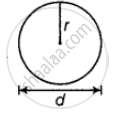# There is a circular pond and a footpath runs along its boundary. A person walks around it, exactly once keeping close to the edge. If his step is 66 cm long and he takes exactly 400 steps to go - Mathematics

Sum

There is a circular pond and a footpath runs along its boundary. A person walks around it, exactly once keeping close to the edge. If his step is 66 cm long and he takes exactly 400 steps to go around the pond, find the diameter of the pond.

#### Solution

Let the radius of the pond be r.

Then, diameter of the pond, d = 2 xx r  ......[∵ Diameter = 2 × radius]Since, a person takes exactly 400 steps with 66 cm long each step to go round the pond.

Hence, the circumference of the pond = 66 x 400 = 26400 cm

= 26400/100

= 264 m  .....[ 100 cm = 1 m]

We know that, circumference of a circle is 2pir.

∴ 2pir = 264

⇒ r = 264/2 xx 7/22

= (264 xx 7)/44

= 42 m

Hence, radius of the pond = 42 m

So, diameter of the pond = 2 xx 42 = 84 m

Concept: Concept of Surface Area, Volume, and Capacity
Is there an error in this question or solution?

#### APPEARS IN

NCERT Mathematics Exemplar Class 8
Chapter 11 Mensuration
Exercise | Q 70 | Page 353